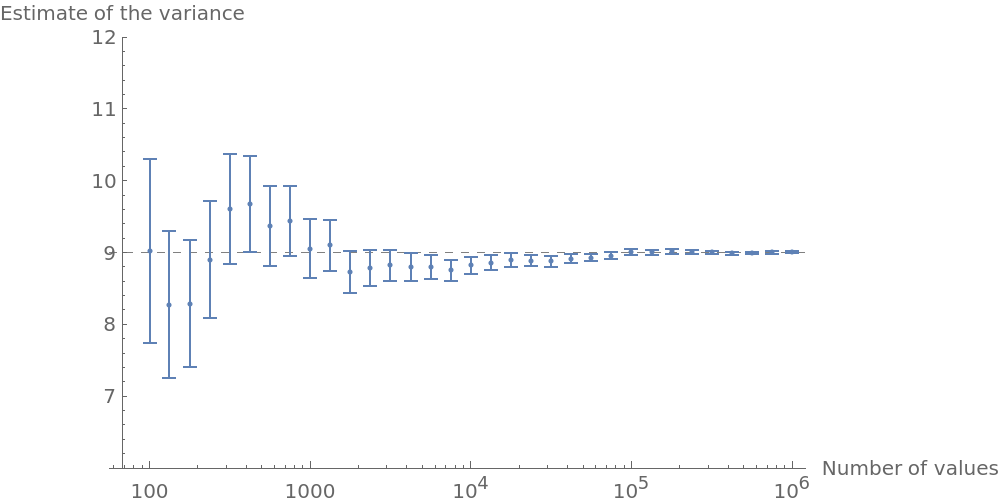Function Repository Resource:

VarianceAround

Calculate the variance of a list along with its uncertainty

Contributed by: Sander Huisman
 ResourceFunction["VarianceAround"][{x1,x2,x3,…}] gives an Around object describing the variance of xi and its uncertainty.

Details and Options

The standard error of the variance is defined as: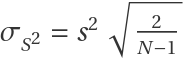,  where s2 represents the variance of the sample.
The “Value” of the Around object is calculated using the built-in Variance function.

Examples

Basic Examples

Calculate the variance of some numbers:

 In:=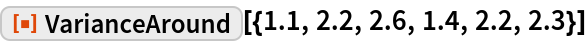Out=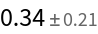Scope

VarianceAround also works with (partially) symbolic expressions:

 In:=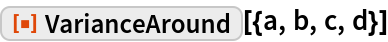Out=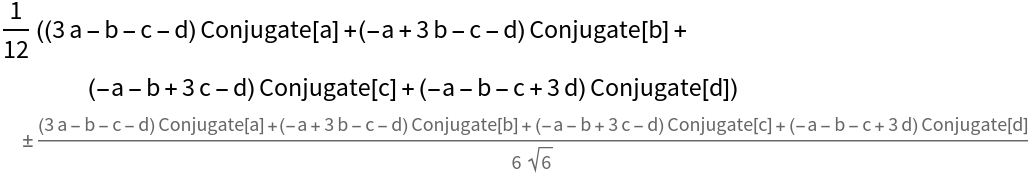VarianceAround also works with quantities with units:

 In:=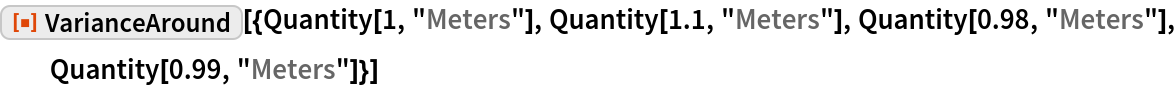Out=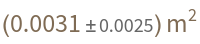Possible Issues

VarianceAround with symbolic expressions can get unwieldy fast:

 In:=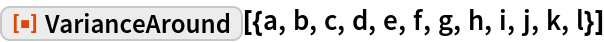Out=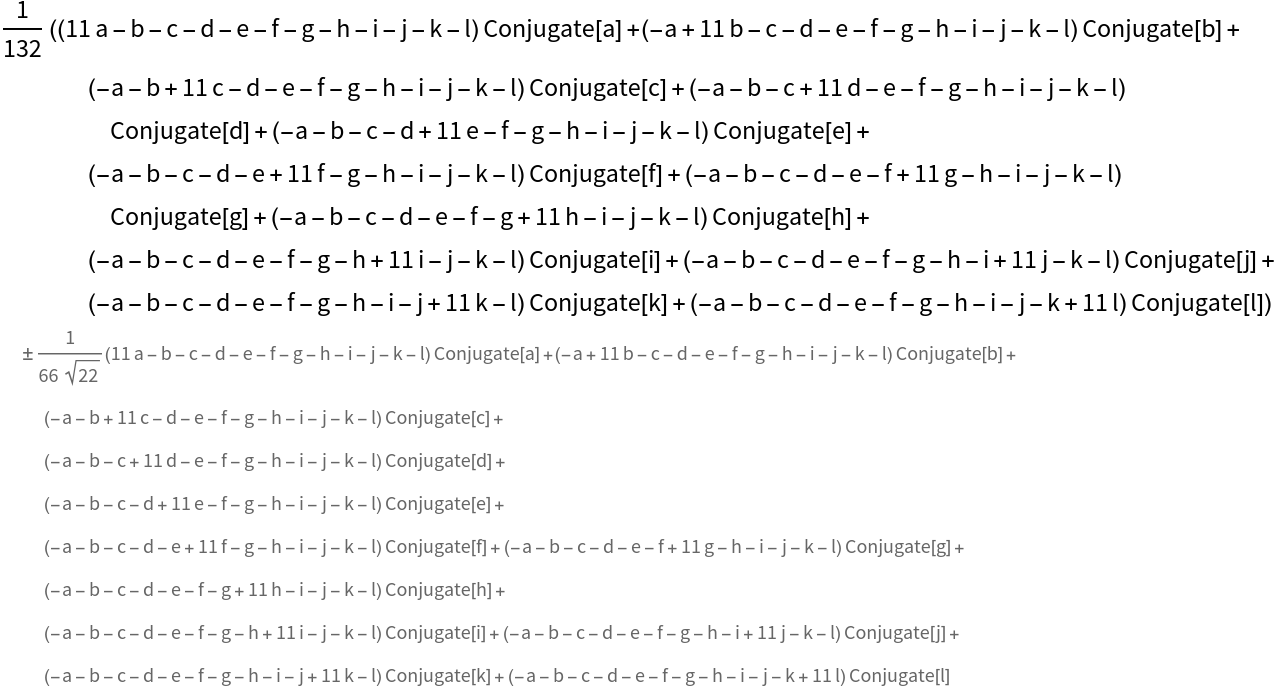The variance cannot be calculated for a list with a single element:

 In:=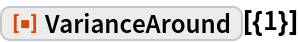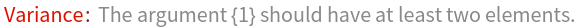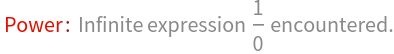Out=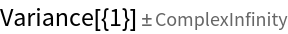Neat Examples

Study the variance and its uncertainty for a sequence of values and compare to the theoretical value of the distribution:

 In:=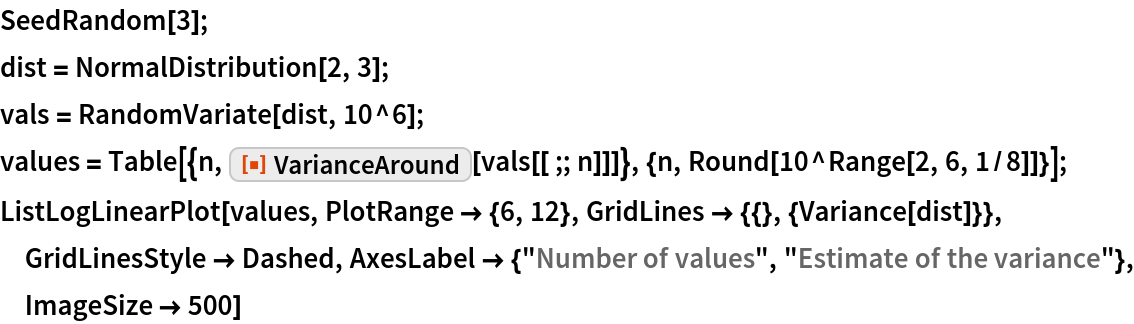Out=